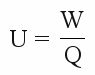# Voltage

## Terminology

At the previous chapter we calculated the amount of energy needed to move two charges with different signs apart from each other respectively the amount of energy being released while those charges approach. The amount of work used respectively the amount of energy converted, is called voltage. The general definition is:
Voltage is a physical quantity, indicating the amount of energy necessary to move a given charge in an electric field.
The symbol used for voltage is U (from the Latin word ugere meaning pushing) and the unit symbol according to the Système international is V. It is named after the Italian physicist Count Alessandro Giuseppe Antonio Anastasio Volta.

## Potential

The electric potential at a point in space is electrical potential energy divided by charge that is associated with a static electric field. Let's have a look at the electric field of a point charge, as we got to know at the previous chapter. While moving a charge from point A to Point B inside this electric field, there is always the same amount of work to be done, independent from the path taken to reach Point B.

Figure 1:
The picture at the left shows the electric field of a positive charge. The significantly smaller test charge is moved from point A to point B inside the field. Because of the fact that both charges have the same sign (+), mechanical work has to be done to move the test charge closer to the main charge. The amount of transformed energy is independent from the path taken. No matter if the test charge is moved directly to point B (blue line), if the green path is taken or even if it is moved away from point B before it goes there (red path): There is always the same amount of work to be done. Let's take an electric car without air or rolling resistance on a mountain instead of the test charge inside an electric field. To be able to drive uphill (=the distance between the two charges decreases), operating power is used to create height energy (=potential energy), because the car is lifted. Because of the fact that there is no air and rolling resistance, there is no difference if the car drives straight upwards or if it is driving around serpentines. If the car is coasting downhill (=the distance between the charges is increased), height energy is converted into kinetic energy. As soon as the car is turning around to drive uphill, the kinetic energy is converted back into height energy and the amount of kinetic energy brings the car back to the same height of point A. From this height you need operating power to lift the car until reaching point B and the amount of the operating power is the same as driving the green or blue path.
The dark red circles mark places of the same potential (same height) and are called equipotential lines. If the test charge is moved along those lines, no work is needed to do this.

The bench mark of the electric potential at the previous chapter was the infinity (=no force, no potential). Now we can define voltage as the difference of two potential points inside an electric field. That is why voltage is also called potential difference. As mathematical expression we get:

[3.3]Where is:
U - Voltage, W - work or transformed energy, Q - charge

## Direction

If an electric field is doing positive work while moving a positive charge from point A to point B, the voltage between point A and B has a positive value. For this reason, at the example given above, the voltage between A and B is negative.